# 一文学会回溯算法解题技巧

danielmou

0收藏

### 前言

​上文​​我们学习了深度优先搜索和广度优先搜索，相信大家对这两者的算法有了比较清楚的认识，值得一提的，深度优先算法用到了回溯的算法思想，这个算法虽然相对比较简单，但很重要，在生产上广泛用在正则表达式，编译原理的语法分析等地方，很多经典的面试题也可以用回溯算法来解决，如八皇后问题，排列组合问题，0-1背包问题，数独问题等，也是一种非常重要的算法。

1. 什么是回溯算法
2. 回溯算法解题通用套路
3. 经典习题讲解

### 回溯算法解题通用套路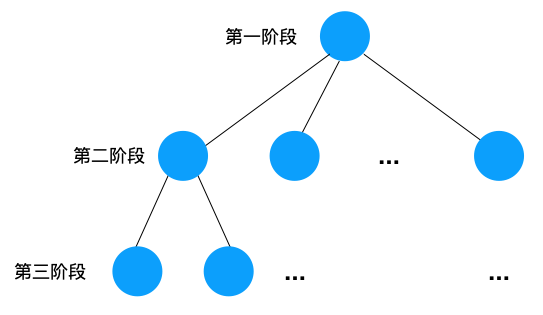1. 如果「已选解集合」满足问题的条件，则将 「已选解集合」放入「结果集」中，并且「回溯」换个解再遍历。
2. 如果不满足，则「回溯」换个解再遍历

``````
function backtrace(已选解集合,每个阶段可选解){
if (已选解集合满足条件) {
return;
}

// 遍历每个阶段的可选解集合
for (可选解 in 每个阶段的可选解) {

// 选择此阶段其中一个解,将其加入到已选解集合中

// 进入下一个阶段
backtrace(已选解集合,下个阶段可选的空间解)

// 「回溯」换个解再遍历
已选解集合.remove(可选解)

}
}``````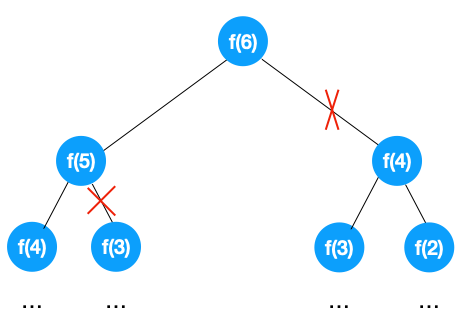1. 将问题分成多个阶段，每个阶段都有多个不同的解，这样就将问题转化成了树形问题，这一步是问题的关键！如果能将问题转成树形问题，其实就成功了一半，需要注意的是树形问题要明确终止条件，这样可以在 DFS 的过程中及时终止遍历，达到剪枝的效果
2. 套用上述回溯算法的解题模板，进行深度优先遍历，直到找到问题的解。

### 经典习题讲解

1、将问题转为树形结构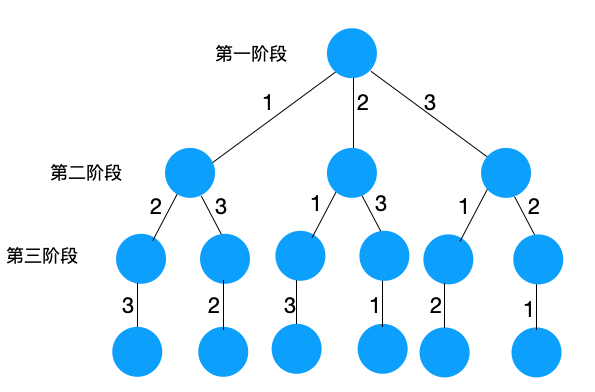2、套用上述回溯算法的解题模板，进行深度优先遍历，直到找到问题的解

``````    public     class Solution {
/**
* 结果集
*/
private     static List<String> RESULT =     new ArrayList<>(10);

/**
* 参与全排列的数字
*/
private     static List<Integer> NUMS = Arrays.asList(1, 2, 3);

/**
* 遍历当前阶段的解
* @param selectedNums   已选解集合
* @param selectableNums 可选的解集合
*/
public     static     void permutation(List<Integer> selectedNums, List<Integer> selectableNums {

// 满足条件，加入结果集
if== NUMS.size()) {
return;
}

// 遍历每个阶段的可选解集合
for (    int i = 0; i < selectableNums.size(); i++) {
Integer num = selectableNums.get(i);

// 去除不符合条件的解，减枝
if (selectedNums.contains(num)) {
continue;
}

// 选择当前阶段其中一个解

// 选完之后再进入下个阶段遍历
permutation(selectedNums, selectableNums);

// 回溯,换一个解继续遍历
selectedNums.remove(num);
}
}

public     static     void main(String[] args){
List<Integer> selectedNums =     new ArrayList<>();
permutation(selectedNums, NUMS);
System.out.println(Arrays.toString(RESULT.toArray()));
}
}``````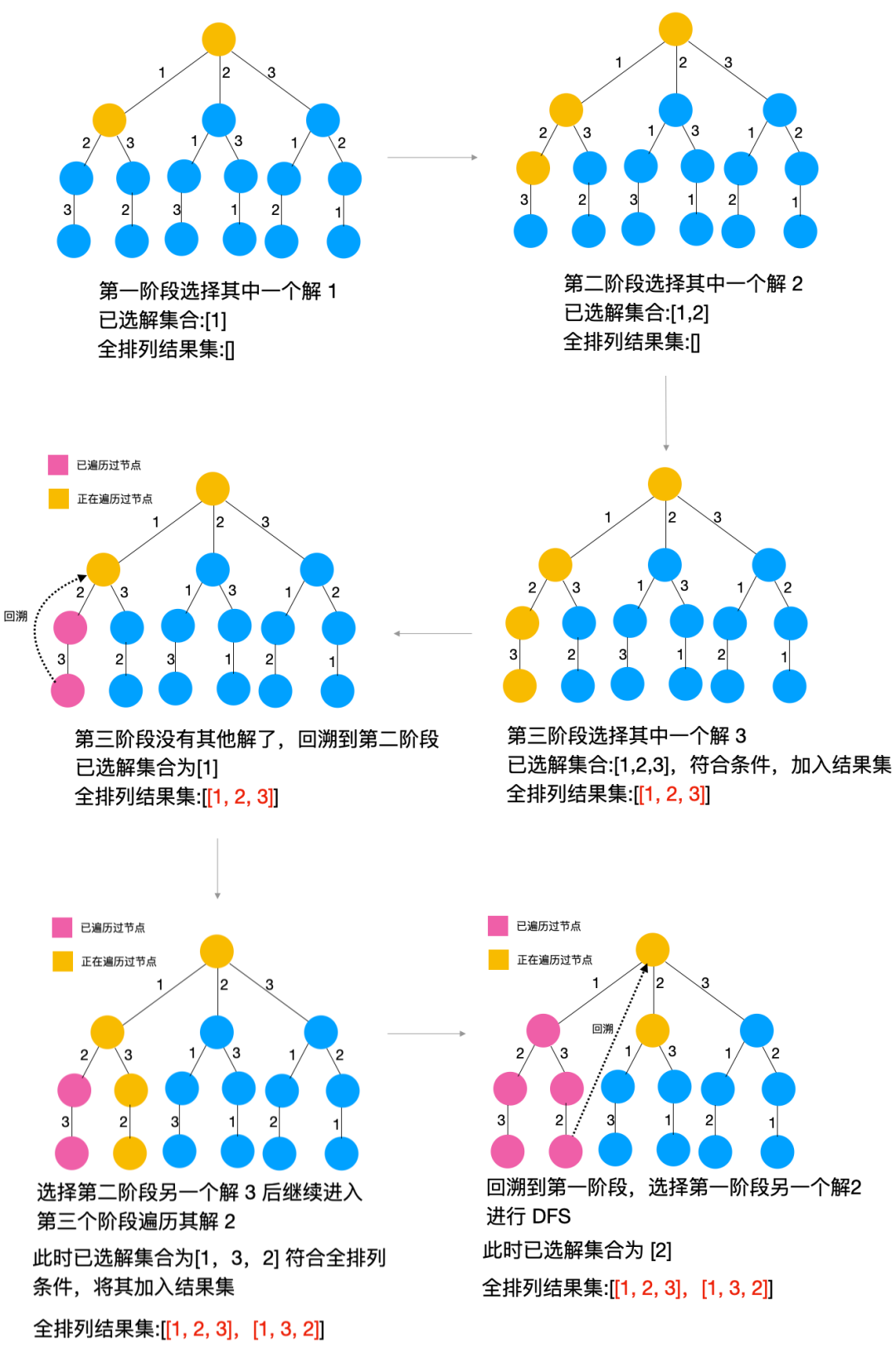1、将问题转为树形结构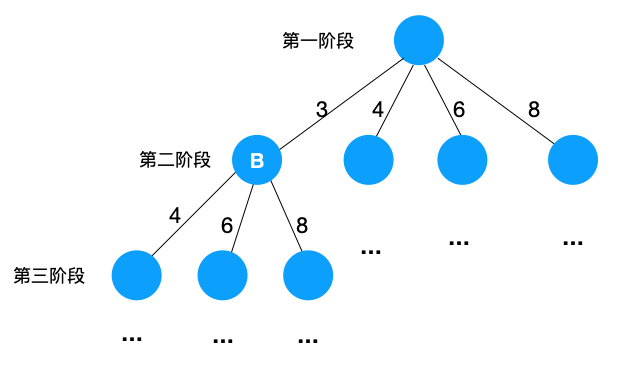2、套用上述回溯算法的解题模板，进行深度优先遍历，直到找到问题的解

``````
public     class Solution {
/**
* 结果集
*/
private     static Integer RESULT = 0;

/**
* 背包最大承载质量
*/
private     static Integer KNAPSACK_MAX_WEIGHT = 10;

/**
* 现有背包
*/
private     static List<Integer> WEIGHTS = Arrays.asList(3, 4, 6, 8);

/**
* 遍历当前阶段的解
*
* @param selectedWeights  已选解集合
* @param selectableWeight 可选的解集合
*/
public     static     void knapsack(List<Integer> selectedWeights, List<Integer> selectableWeight){

{
// 求已选物品的总重量
int sumOfWeights = selectedWeights.stream().mapToInt(Integer::intValue).sum();
if (sumOfWeights == KNAPSACK_MAX_WEIGHT) {
RESULT = Math.max(RESULT, sumOfWeights);
return;
}     else     if (sumOfWeights > KNAPSACK_MAX_WEIGHT) {
// 如果已选物品的总重量超过背包最大承受质量，则要把最后一个选择的物品移除，再求质量和
selectedWeights.remove(selectedWeights.size() - 1);
sumOfWeights = selectedWeights.stream().mapToInt(Integer::intValue).sum();
RESULT = Math.max(RESULT, sumOfWeights);
return;
}     else {
RESULT = Math.max(RESULT, sumOfWeights);
}
}

// 遍历每个阶段的可选解集合
for (    int i = 0; i < selectableWeight.size(); i++) {
Integer num = selectableWeight.get(i);

// 去除不符合条件的解，减枝
if (selectedWeights.contains(num)) {
continue;
}

// 选择子节点的其中一个解

// 选完之后再进行 dfs
knapsack(selectedWeights, selectableWeight);

// 「回溯」换个解再遍历
selectedWeights.remove(num);
}

}

public     static     void main(String[] args){
List<Integer> selectedNums =     new ArrayList<>();
knapsack(selectedNums, WEIGHTS);
System.out.println("result = " + RESULT);
}
}
``````

3、八皇后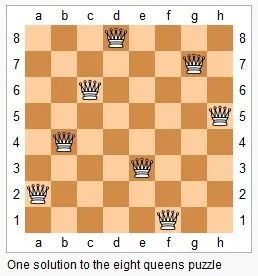1、将问题转为树形结构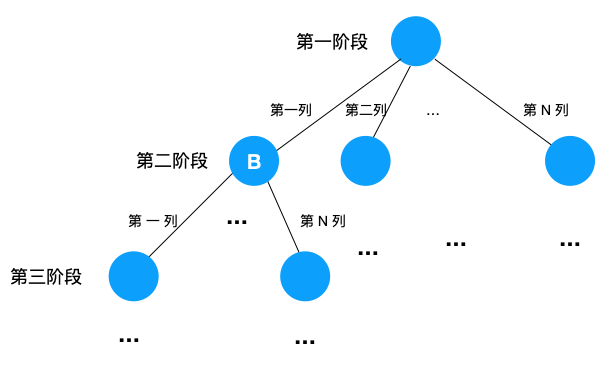2、套用上述回溯算法的解题模板，进行深度优先遍历，直到找到问题的解

``````    public     class Solution {

private     static Integer N = 8;

/**
*
* @param selectedColumns 已选解集合,下标表示行,值表示queen存储在哪一列
* @param row             可选的空间解,第 n 行可选
*/
public     static     void queenSettle(    int[] selectedColumns,     int{
// 终止条件
if (row > N - 1) {
// 说明前 N 行都已经都选完皇后了，
printQueens(selectedColumns);
return;
}

for (    int i = 0; i < N; i ++) {

// 剔除不合法的格子
if (!isValid(row, i, selectedColumns)) {
continue;
}

// 选择子节点（当前行）其中一个解
selectedColumns[row] = i;

// 选完之后再进入下个阶段的（下一行）遍历
queenSettle(selectedColumns, row + 1);

// 回溯,换一个解继续 dfs，回溯时要把回溯节点的解移除
selectedColumns[row] = -1;

}
}

/**
* 判断相应的格子放置皇后是否OK
* @param row
* @param column
* @param selectedColumns
* @return
*/
private     static     boolean isValid(    int row,     int column,     int[] selectedColumns){
//判断row行column列放置是否合适
int leftup = column - 1, rightup = column + 1;
for (    int i = row-1; i >= 0; --i) { // 逐行往上考察每一行
if (selectedColumns[i] == column)     return     false; // 第i行的column列有棋子吗？
if (leftup >= 0) { // 考察左上对角线：第i行leftup列有棋子吗？
if (selectedColumns[i] == leftup)     return     false;
}
if (rightup < 8) { // 考察右上对角线：第i行rightup列有棋子吗？
if (selectedColumns[i] == rightup)     return     false;
}
--leftup; ++rightup;
}
return     true;
}

public     static     void main(String[] args){
int[] selectedColumn =     new     int[N];
// 从第 0 行开始 DFS
queenSettle(selectedColumn, 0);
}

private     static     void printQueens(    int[] result){ // 打印出一个二维矩阵
for (    int row = 0; row < 8; ++row) {
for (    int column = 0; column < 8; ++column) {
if (result[row] == column) System.out.print("Q ");
else System.out.print("* ");
}
System.out.println();
}
System.out.println();
}
}``````

### 总结